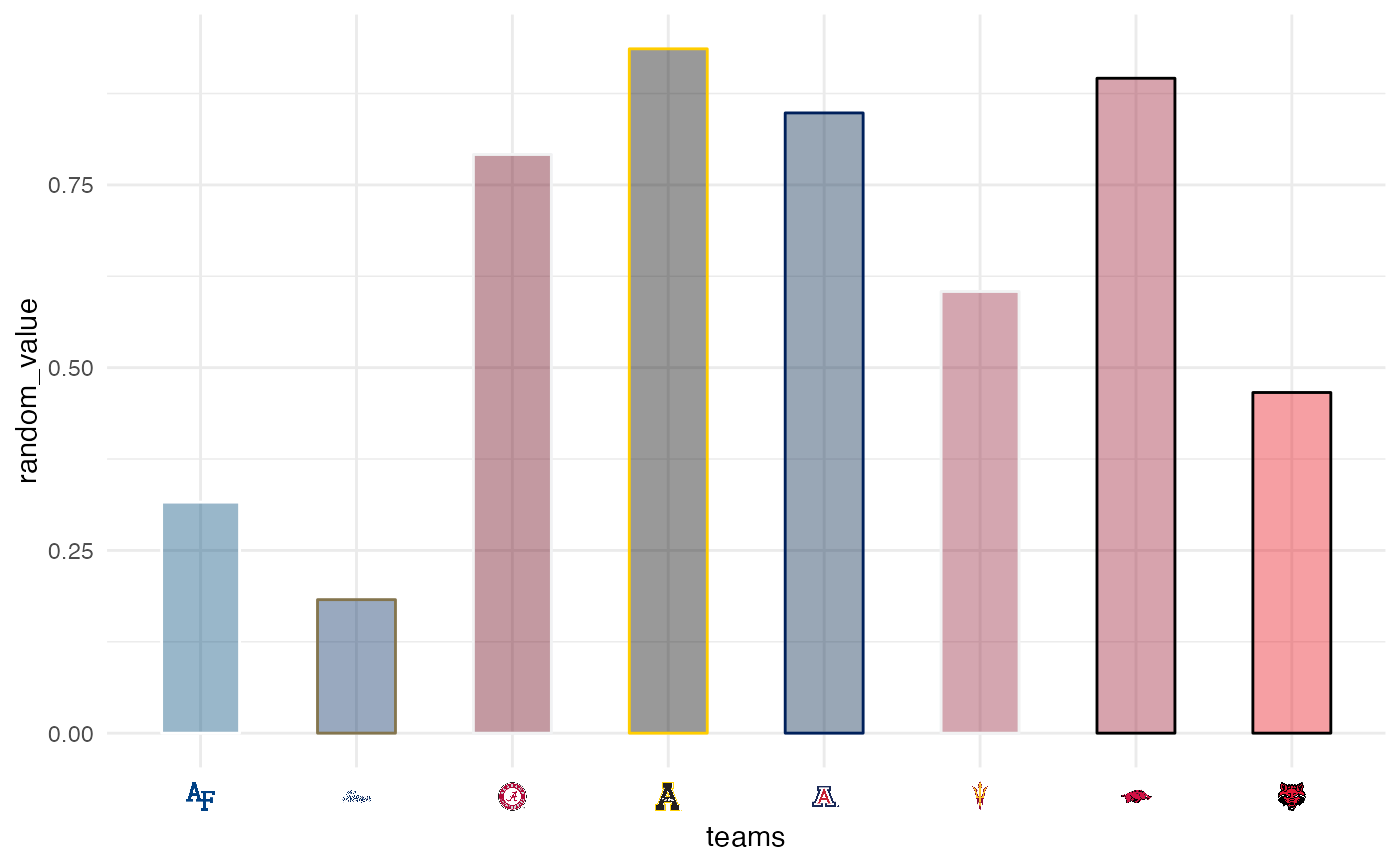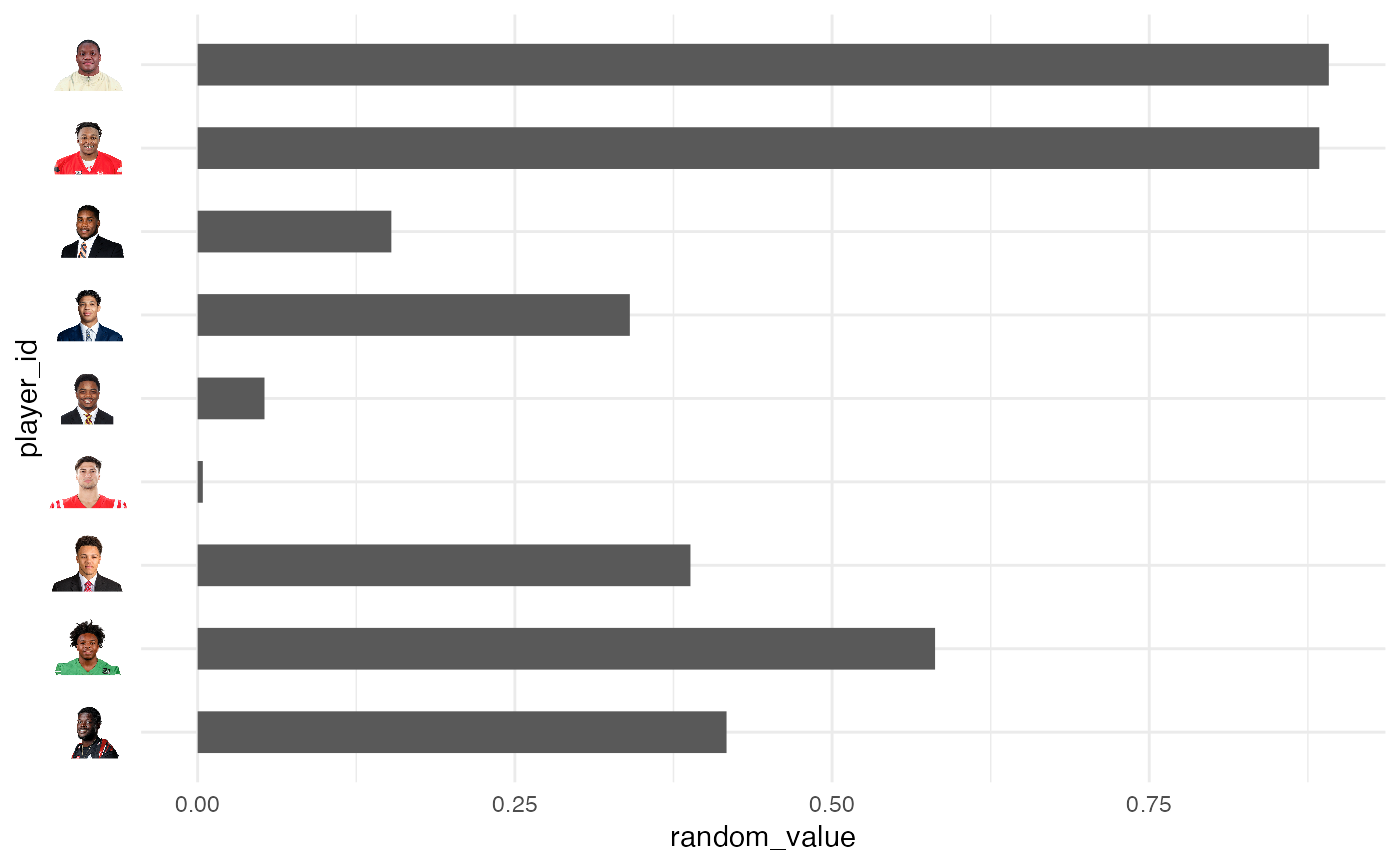These functions map CFB team names to their team logos and make them available as axis labels

scale_x_cfb(
...,
expand = ggplot2::waiver(),
guide = ggplot2::waiver(),
position = "bottom",
size = 12
)

scale_y_cfb(
...,
expand = ggplot2::waiver(),
guide = ggplot2::waiver(),
position = "left",
size = 12
)

...,
expand = ggplot2::waiver(),
guide = ggplot2::waiver(),
position = "bottom",
size = 20
)

...,
expand = ggplot2::waiver(),
guide = ggplot2::waiver(),
position = "left",
size = 30
)

## Arguments

...

Arguments passed on to discrete_scale

palette

A palette function that when called with a single integer argument (the number of levels in the scale) returns the values that they should take (e.g., scales::hue_pal()).

breaks

One of:

• NULL for no breaks

• waiver() for the default breaks (the scale limits)

• A character vector of breaks

• A function that takes the limits as input and returns breaks as output. Also accepts rlang lambda function notation.

limits

One of:

• NULL to use the default scale values

• A character vector that defines possible values of the scale and their order

• A function that accepts the existing (automatic) values and returns new ones. Also accepts rlang lambda function notation.

drop

Should unused factor levels be omitted from the scale? The default, TRUE, uses the levels that appear in the data; FALSE uses all the levels in the factor.

na.translate

Unlike continuous scales, discrete scales can easily show missing values, and do so by default. If you want to remove missing values from a discrete scale, specify na.translate = FALSE.

na.value

If na.translate = TRUE, what aesthetic value should the missing values be displayed as? Does not apply to position scales where NA is always placed at the far right.

aesthetics

The names of the aesthetics that this scale works with.

scale_name

The name of the scale that should be used for error messages associated with this scale.

name

The name of the scale. Used as the axis or legend title. If waiver(), the default, the name of the scale is taken from the first mapping used for that aesthetic. If NULL, the legend title will be omitted.

labels

One of:

• NULL for no labels

• waiver() for the default labels computed by the transformation object

• A character vector giving labels (must be same length as breaks)

• A function that takes the breaks as input and returns labels as output. Also accepts rlang lambda function notation.

super

The super class to use for the constructed scale

expand

For position scales, a vector of range expansion constants used to add some padding around the data to ensure that they are placed some distance away from the axes. Use the convenience function expansion() to generate the values for the expand argument. The defaults are to expand the scale by 5% on each side for continuous variables, and by 0.6 units on each side for discrete variables.

guide

A function used to create a guide or its name. See guides() for more information.

position

For position scales, The position of the axis. left or right for y axes, top or bottom for x axes.

size

The logo size in pixels. It is applied as height for an x-scale and as width for an y-scale.

## Details

The scale translates the CFB team names into raw image html and places the html as axis labels. Because of the way ggplots are constructed, it is necessary to adjust the theme() after calling this scale. This can be done by calling theme_x_cfb() or theme_y_cfb() or alternatively by manually changing the relevant axis.text to ggtext::element_markdown().

theme_x_cfb(), theme_y_cfb()

## Examples

library(cfbplotR)
library(ggplot2)

team_abbr <- cfbplotR::valid_team_names()
# remove conference logos from this example
team_abbr <- team_abbr[1:8]

df <- data.frame(
random_value = runif(length(team_abbr), 0, 1),
teams = team_abbr
)
if (utils::packageVersion("gridtext") > "0.1.4"){
# use logos for x-axis
ggplot(df, aes(x = teams, y = random_value)) +
geom_col(aes(color = teams, fill = teams), width = 0.5) +
scale_color_cfb(alt_colors = team_abbr) +
scale_fill_cfb(alpha = 0.4) +
scale_x_cfb() +
theme_minimal() +
# theme_*_cfb requires gridtext version > 0.1.4
theme_x_cfb()
}#############################################################################
#############################################################################
library(cfbplotR)
library(ggplot2)

dfh <- data.frame(
random_value = runif(9, 0, 1),
player_id = c("4361182",
"4426385",
"4567048",
"4372519",
"4429013",
"4240069",
"4360932",
"4362874",
"4429299")
)
if (utils::packageVersion("gridtext") > "0.1.4"){
# use headshots for x-axis
ggplot(dfh, aes(x = player_id, y = random_value)) +
geom_col(width = 0.5) +
theme_minimal() +
# theme_*_cfb requires gridtext version > 0.1.4
theme_x_cfb()

# use headshots for y-axis
ggplot(dfh, aes(y = player_id, x = random_value)) +
geom_col(width = 0.5) +